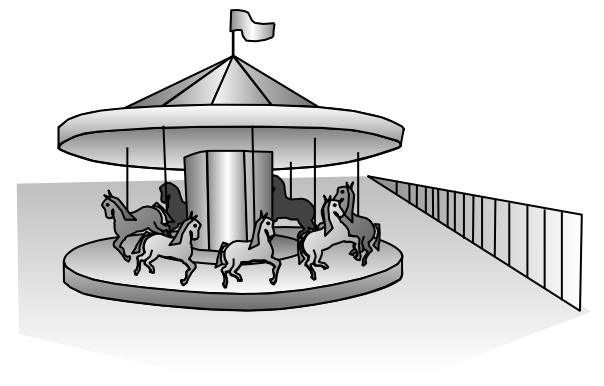### Home > PC3 > Chapter 8 > Lesson 8.1.2 > Problem8-26

8-26.

Jade is having a wonderful time riding a carousel. Her distance, $d$ (in feet), from the fence nearest the entrance is given by the equation $d=25\sin\left(\frac{\pi t}{15}\right)+35$, where $t$ is the time in seconds since the carousel started to rotate.1. How close can Jade be to the fence? How far?

What are the minimum and maximum values of the function?

2. How much time does it take for the carousel to complete one revolution?

This is the time for one cycle, or the period.
period $=\frac{2\pi}{b}$

3. When is Jade $60$ feet from the fence for the second time?

Solve $f(t)=60$.

4. Sketch the graph of this situation. Label and scale the axes appropriately.